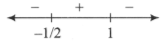Monotonicity
Question

# If $\mathrm{f}\left(\mathrm{x}\right)={\mathrm{xe}}^{\mathrm{x}\left(\mathrm{x}-1\right)}$, then f(x) is

Easy
Solution

## $\mathrm{f}\left(\mathrm{x}\right)={\mathrm{xe}}^{\mathrm{x}\left(1-\mathrm{x}\right)}$or     Sign scheme of f '(x) is as follows:Thus, g(x) is increasing in [-½,1].

Get Instant Solutions